Next: The Photon Propagator Up: Photons Previous: Photons

## Polarization Vectors

Turning now from classical mechanics to quantum mechanics, a free photon can be represented by a wave function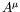, which satisfies the equation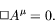(7.97)

A plane-wave solution to this equation is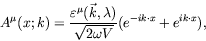(7.98)

where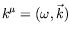is the four-momentum and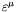is the unit polarization four-vector of the photon. We will discuss the polarization and normalization presently.

On substituting into the equation, we fine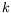satisfies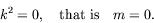(7.99)

The polarization vector has four components and yet it describes a spin-1 particle. The Lorentz condition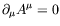, gives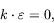(7.100)

and this reduces the number of independent components ofto three. Moreover, we have to explore the consequences of the additional gauge freedom. Choose a gauge parameter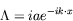(7.101)

with constant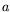so that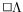is satisfied. This, together with the solution forshows that the physics is unchanged by the replacement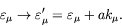(7.102)

In other words, two polarization vectors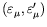which differ by a multiple of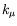describe the same photon. We may use this freedom to ensure that the time component ofvanishes,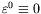; and then the Lorentz condition reduces to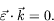(7.103)

This (noncovariant) choice of gauge is known as the Coulomb gauge.

We see that there are only two independent polarization vectors and that they are both transverse to the three-momentum of the photon. For example, for a photon traveling along the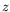-axis, we may take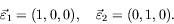(7.104)

These are referred to as linear polarization vectors. The linear combinations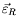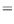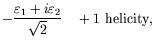(7.105)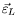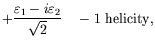(7.106)

are called circular polarization vectors. A free photon is thus described by its momentum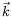and a polarization vector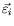. Sincetransforms as a vector, we anticipate that it is associated with a particle of spin-1. If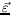were along, it would be associated with a helicity-zero photon. This state is missing because of the transversability condition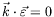. It can only be absent because the photon is massless.

In a special Lorentz frame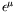is pure space-like,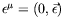, with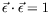. In an arbitrary Lorentz frameis space-like and normalized to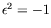. The normalization constant of the plane wave for a photon is chosen such that the energy in the waveis just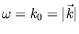(ie.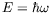for a single photon). To verify this, we compute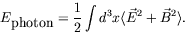(7.107)

This if for the Heaviside-Lorentz system of units. In the Gaussian system of units the constant in front of the integral would be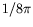. Since (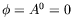in the Lorentz gauge)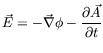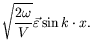(7.108)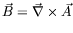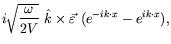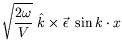(7.109)

and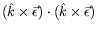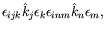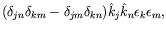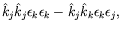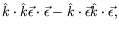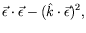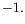(7.110)

Thus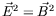and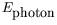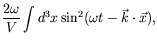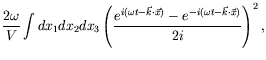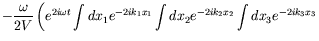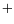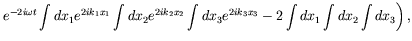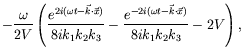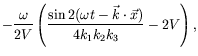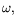(7.111)

where we have taken the time average.

The completeness relationship for polarization vectors is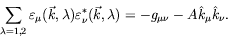(7.112)Next: The Photon Propagator Up: Photons Previous: Photons
Douglas M. Gingrich (gingrich@ ualberta.ca)
2004-03-18